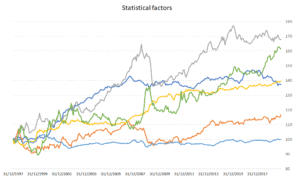# Statistical factor models

Statistical factor models are used by investment professionals to model asset returns. They are one of three kinds of factors models. The two other models are macroeconomic factor models and fundamental factor models

Interestingly, statistical factor models are both easier and more difficult to apply than the other two types of models, for different reasons. On the one hand, far less data is needed to estimate a statistical factor model. This makes it very easy to collect the necessary data. On the other hand, the statistical methods themselves are somewhat more difficult to implement, at least using a simple spreadsheet. This means it is hard to create a statistical factor model example without using software such as Python or MATLAB.

On this page, we provide a primer on statistical factor models and discuss the methods professional use to estimate statistical factor models.

## Statistical factor model types

There are two primary methods that are used to create statistical factors models. Theses methods are factor analysis and principal component analysis. In factor analysis, factors are portfolios that explain the covariance in asset returns. In principal component models, the factors are portfolios that explain the variance in asset returns.